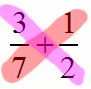# Learn to add two fractions together

Since you're just learning fractions, they probably seem really complicated right now. Remember, though, that (at this point) a fraction is just a number, and so they can be added, subtracted, multiplied, and divided. Fortunately, adding two fractions is not nearly as difficult as you might think. Here's a really simple example to get us started:

### Example:

$$\frac{1}{3}+\frac{1}{3}=?$$

How can we add these two fractions? Since they have a "common denominator" (the bottom number is the same), we can just add the top numbers together like this:

$$\frac{1}{3}+\frac{1}{3}=\frac{2}{3}$$

Sadly, all fractions won't be this easy to add. In order to add two fractions, you must first find a common denominator. We can't just add 1/3 to 1/5 because they don't have the same denominator. The answer is most definitely NOT 2/8, so don't make that mistake.

Let's suppose we get a problem like this: $$\frac{1}{3} + \frac{1}{6} = ?$$. How do we solve that if we can't just add the numerators (the top numbers)? What number should go on the bottom? Well, the trick is to rewrite one or both of the fractions so they have the same denominator! Isn't $$\frac{1}{3}$$ really the same as $$\frac{2}{6}$$ afterall?

$$\frac{1}{3}+\frac{1}{6}$$

Since 1/3 = 2/6, we can substitute without changing the value:

$$\frac{2}{6}+\frac{1}{6}$$

Now we can just add the numerators (top numbers) together:

$$\frac{2+1}{6}$$ $$\frac{3}{6}$$ $$\frac{1}{2}$$

What if it isn't so simple to find a common denominator? One way to find a common denominator is to multiply the two denominators and use that. In the previous example, that would mean using 18 as the common denominator. Here's an example:

### Example finding common denominator

$$\frac{1}{4}+\frac{2}{5}$$

What common denominator can we use? We can't just change one of the denominators to make it equal the other one, so we'll have to change both. We'll find one by multiplying the denominators together. When we multiply $$4*5$$ we get a common denominator of 20. So, we need to rewrite both fractions with 20 in the bottom. We'll do that by multiplying $$\frac{1}{4}*\frac{5}{5}$$ and then by multiplying $$\frac{2}{5}*\frac{4}{4}$$. Since we're multiply the fractions by terms equivalent to 1, we haven't altered the value of anything -- we've just rewritten our fractions to have a common denominator.

$$\frac{1*5}{4*5}+\frac{2*4}{5*4}$$ $$\frac{5}{20}+\frac{8}{20}$$ $$\frac{5+8}{20}$$ $$\frac{13}{20}$$

Is there an easier method to add fractions with different denominators? You bet. It's called cross-multiplying, and it's really just an automated version of what we just did. First, you multiply the top number on the left by the bottom number on the right, and top number on the right by the bottom number on the left. See why it's called cross-multiplying? The image below shows how you might start adding $$\frac{3}{7}+\frac{1}{2}$$ by cross-multiplying $$3*2$$ and $$7*1$$. After that, you add the two results.In the above problem, after we multiply $$3*2$$ and $$7*1$$, we add the results. That gives us $$6+7$$, which equals $$13$$. That will be the top number of the answer! To get the bottom number, just multiply the bottom numbers together and that's all. So the final answer is $$13/14$$.

Here's a few more examples where I cross multiply as a way of adding fractions:

$$\frac{1}{4}+\frac{2}{5}=\frac{1*5+2*4}{4*5}=\frac{5+8}{20}=\frac{13}{20}$$ $$\frac{4}{9}+\frac{4}{5}=\frac{4*5+4*9}{9*5}=\frac{20+36}{45}=\frac{56}{45}$$ $$\frac{1}{11}+\frac{1}{2}=\frac{1*2+1*11}{11*2}=\frac{2+11}{22}=\frac{13}{22}$$